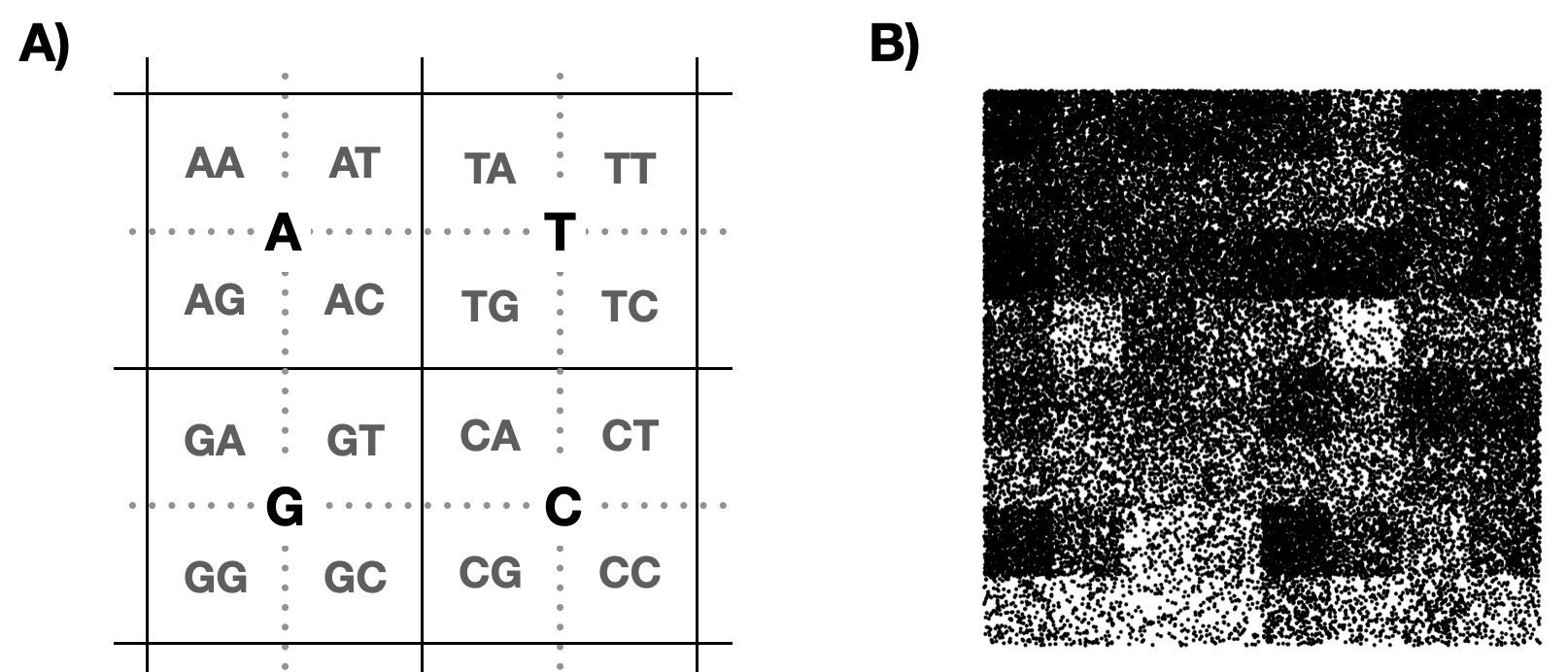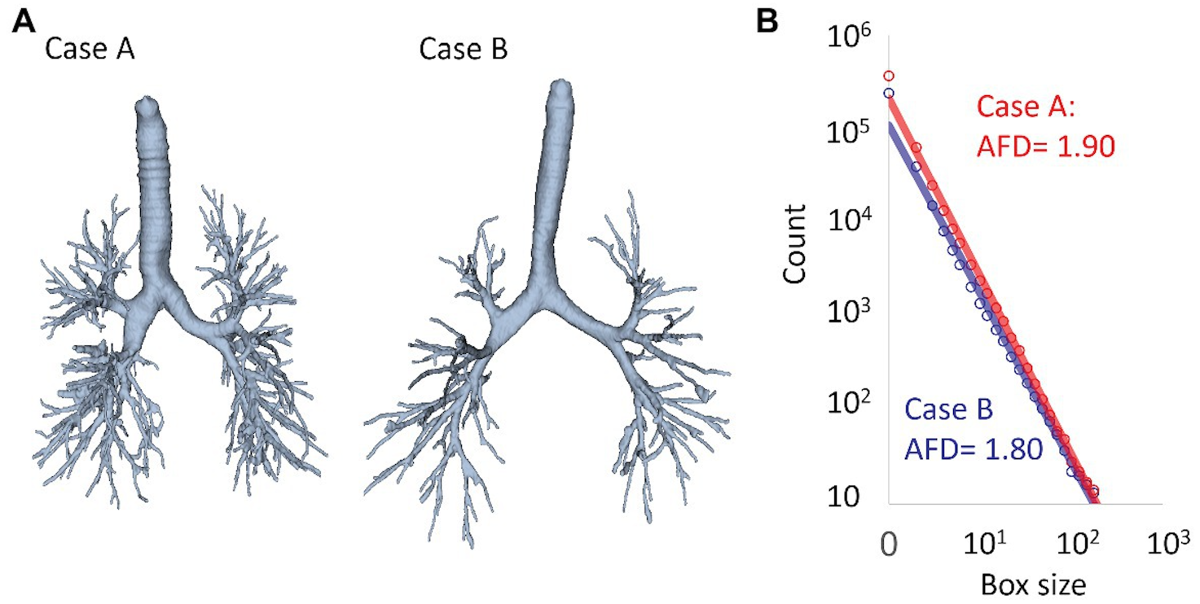## 3.3 Applications

### 3.3.1 Chaos game and genetics

Dear Reader, take a moment to consider the implications of the last example. By choosing different probabilities, you get visually different figures. At infinity, the entire fractal would saturate, but with a finite number of steps, the density of points encodes information about the distribution of the drawn transformations.

This observation has led to a number of interesting applications of the chaos game to encode information in sequences of varying lengths. It turns out that we can “wind” strings of seemingly random symbols onto fractals so that we can visually examine the relative frequencies of these symbols.

Let’s look at applying this idea to encoding genetic sequences. The sequences consist of strings of the letters A, C, T and G. Let’s consider four transformations that are based on a double reduction and shifting toward one of the corners of the square.Chaos game on biological sequences. The left panel illustrates the method by which the encoding works. The right panel encodes the genetic sequence of the SARS-CoV-2 virus

Now, instead of randomly choosing a transformation, we can read some genetic sequence and choose a transformation depending on the letter or sequence of letters encountered. This way, frequently occurring patterns in the sequence will be visually encoded in the fractal.

The example above encodes the sequence of the SARS-CoV-2 virus using four transformations. Other ideas for encoding biological sequences using the chaos game can be found in the article Chaos game representation and its applications in bioinformatics [LöHe21].

### 3.3.2 Chaos game and music

Applications of fractal mathematics have also been sought in music. In the literature, both scientific and popular science, one can find numerous examples of various attempts to combine music with fractals. These range from using fractals to generate music that is neither too monotonous nor too chaotic, to using fractals to study the predictability of various musical genres [K.Hs91].

For example, in 2012, the popular magazine Wired presented a fractal analysis of various musical pieces. They were first described in the language of harmonic components, and then a fractal dimension analysis was performed on them, finding that symphonies were the most predictable, while ragtime pieces were the least predictable.

### 3.3.3 The fractal dimension of the brain

In the previous chapter, we presented the definition of box-counting dimension. Those who assume that it will only be used for fractals would be mistaken. Practitioners have found many interesting applications for it, such as measuring the degree of jaggedness of the coastline of different countries. For example, the box dimension of Australia’s coastline is 1.143 [HRBS21], which indicates a high degree of irregularity. However, this is much less than the dimension of Norway’s very jagged coastline, which is 1.3727.

It was also possible to measure the fractal dimensions of anatomical objects, such as the brain and lungs. It turns out that the surface of the brain has a dimension of 2.79, which is evidence of the large undulation of this organ. The surface of the lungs has an even higher dimension, reaching a value of 2.97 source [Wiki22]. In the medical literature, one can find examples of analyses using the fractal dimension of specific anatomical structures in disease detection.

Below is a figure from an article Fractal Analysis of Lung Structure in Chronic Obstructive Pulmonary Disease [TSSH20] on the use of fractal dimension in the analysis of chronic lung disease. Chronic diseases can lead to changes in anatomical structures that can be quantitatively described by fractal dimension. This helps both to understand the changes caused by the disease and to build predictive models to support diagnosis.Graph from the paper Fractal analysis of lung structure in chronic obstructive pulmonary disease, exploring the possibility of using the fractal dimension of lung blood vessels in the analysis of chronic lung disease. Panel A shows schematic structures of major blood vessels, panel B shows how the number of boxes increases with decreasing size of the side of the box.

Where else can fractal geometry be applied? Surely there are many more interesting discoveries ahead.

### Bibliography

[HRBS21]
Husain, Akhlaq ; Reddy, Jaideep ; Bisht, Deepika ; Sajid, Mohammad: Fractal dimension of coastline of Australia. In: Scientific Reports (2021)
[K.Hs91]
K. J. Hsü ; Hsü, A.: Self-similarity of the ,,1/f noise” called music. In: Proceedings of the National Academy of Sciences Bd. 88 (1991), Nr. 8, S. 3507–3509
[LöHe21]
Löchel, Hannah Franziska ; Heider, Dominik: Chaos game representation and its applications in bioinformatics. In: Computational and Structural Biotechnology Journal Bd. 19 (2021), S. 6263–6271
[TSSH20]
Tanabe, Naoya ; Sato, Susumu ; Suki, Béla ; Hirai, Toyohiro: Fractal Analysis of Lung Structure in Chronic Obstructive Pulmonary Disease. In: Frontiers in Physiology Bd. 11 (2020)
[Wiki22]